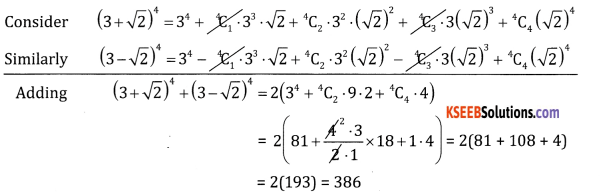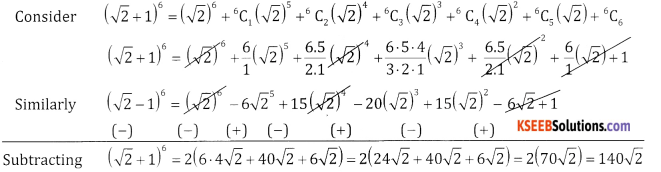# 2nd PUC Basic Maths Question Bank Chapter 4 Binomial Theorem Ex 4.1

Students can Download Basic Maths Exercise 4.1 Questions and Answers, Notes Pdf, 2nd PUC Basic Maths Question Bank with Answers helps you to revise the complete Karnataka State Board Syllabus and score more marks in your examinations.

## Karnataka 2nd PUC Basic Maths Question Bank Chapter 4 Binomial Theorem Ex 4.1

Part – A

2nd PUC Basic Maths Binomial Theorem Ex 4.1 One Mark Questions and Answers

Question 1.
(1 – 2x)5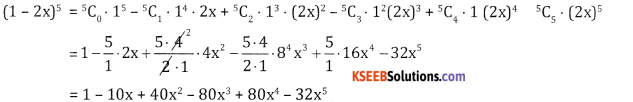Question 2.
( $$\frac{2}{x}-\frac{x}{2}$$ )5Question 3.
(2x – 3)6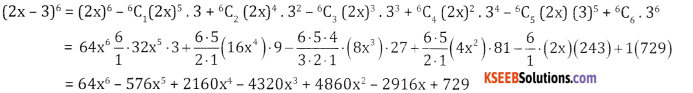Question 4.
$$\left(\frac{x}{3}+\frac{1}{x}\right)^{5}$$Question 5.
$$\left(x+\frac{1}{x}\right)^{6}$$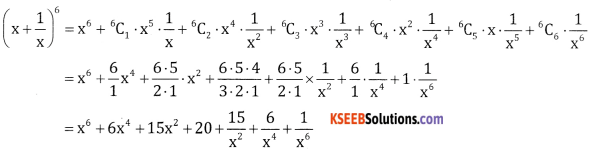Question 6.
$$\left(x^{2}+\frac{3}{x}\right)^{4}$$Part B

2nd PUC Basic Maths Binomial Theorem Ex 4.1 Five Marks Questions and Answers

Question 1.
(√3 + 1)5 – (√3 – 1)5Question 2.
((1 + √5)5 – (1 – √5)5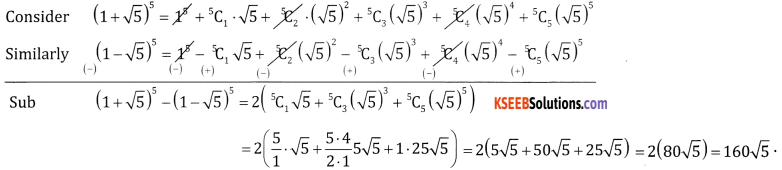Question 3.
(3 + √2)4 + (1 – √2)4